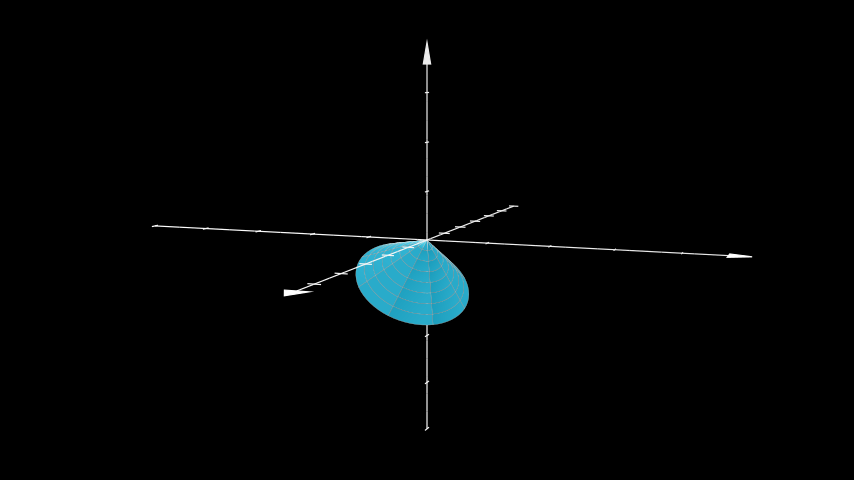# Cone#

Qualified name: `manim.mobject.three\_d.three\_dimensions.Cone`

class Cone(base_radius=1, height=1, direction=array([0., 0., 1.]), show_base=False, v_range=[0, 6.283185307179586], u_min=0, checkerboard_colors=False, **kwargs)[source]#

Bases: `Surface`

A circular cone. Can be defined using 2 parameters: its height, and its base radius. The polar angle, theta, can be calculated using arctan(base_radius / height) The spherical radius, r, is calculated using the pythagorean theorem.

Parameters:
• base_radius (float) – The base radius from which the cone tapers.

• height (float) – The height measured from the plane formed by the base_radius to the apex of the cone.

• direction (np.ndarray) – The direction of the apex.

• show_base (bool) – Whether to show the base plane or not.

• v_range (Sequence[float]) – The azimuthal angle to start and end at.

• u_min (float) – The radius at the apex.

• checkerboard_colors (bool) – Show checkerboard grid texture on the cone.

• kwargs (Any) –

Examples

Example: ExampleCone```from manim import *

class ExampleCone(ThreeDScene):
def construct(self):
axes = ThreeDAxes()
cone = Cone(direction=X_AXIS+Y_AXIS+2*Z_AXIS, resolution=8)
self.set_camera_orientation(phi=5*PI/11, theta=PI/9)
```
```class ExampleCone(ThreeDScene):
def construct(self):
axes = ThreeDAxes()
cone = Cone(direction=X_AXIS+Y_AXIS+2*Z_AXIS, resolution=8)
self.set_camera_orientation(phi=5*PI/11, theta=PI/9)

```

Methods

 `func` Converts from spherical coordinates to cartesian. `get_direction` Returns the current direction of the apex of the `Cone`. `set_direction` Changes the direction of the apex of the `Cone`.

Attributes

 `animate` Used to animate the application of any method of `self`. `animation_overrides` `color` `depth` The depth of the mobject. `fill_color` If there are multiple colors (for gradient) this returns the first one `height` The height of the mobject. `n_points_per_curve` `sheen_factor` `stroke_color` `width` The width of the mobject.
_original__init__(base_radius=1, height=1, direction=array([0., 0., 1.]), show_base=False, v_range=[0, 6.283185307179586], u_min=0, checkerboard_colors=False, **kwargs)#

Initialize self. See help(type(self)) for accurate signature.

Parameters:

• height (float) –

• direction (ndarray) –

• show_base (bool) –

• v_range (Sequence[float]) –

• u_min (float) –

• checkerboard_colors (bool) –

• kwargs (Any) –

Return type:

None

func(u, v)[source]#

Converts from spherical coordinates to cartesian.

Parameters:
• u (float) – The radius.

• v (float) – The azimuthal angle.

Returns:

Points defining the `Cone`.

Return type:

`numpy.array`

get_direction()[source]#

Returns the current direction of the apex of the `Cone`.

Returns:

direction – The direction of the apex.

Return type:

`numpy.array`

set_direction(direction)[source]#

Changes the direction of the apex of the `Cone`.

Parameters:

direction (ndarray) – The direction of the apex.

Return type:

None